Next: Plane polar coordinates Up: Keplerian orbits Previous: Kepler's laws

# Conservation laws

As we have already seen, gravity is a conservative force. Hence, the gravitational force in Equation (4.1) can be written (see Section 2.4)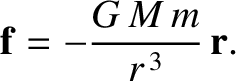(4.3)

where the potential energy,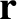, of our planet in the Sun's gravitational field takes the form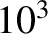(4.4)

(See Section 3.5.) It follows that the total energy of our planet is a conserved quantity. (See Section 2.4.) In other words,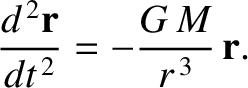(4.5)

is constant in time. Here,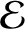is actually the planet's total energy per unit mass, and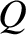.

Gravity is also a central force. Hence, the angular momentum of our planet is a conserved quantity. (See Section 2.5.) In other words,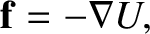(4.6)

which is actually the planet's angular momentum per unit mass, is constant in time. Assuming that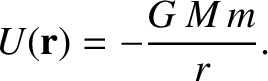, and taking the scalar product of the preceding equation with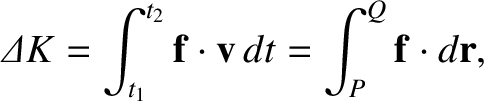, we obtain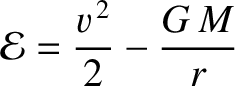(4.7)

This is the equation of a plane that passes through the origin, and whose normal is parallel to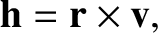. Becauseis a constant vector, it always points in the same direction. We, therefore, conclude that the orbit of our planet is two-dimensional; that is, it is confined to some fixed plane that passes through the origin. Without loss of generality, we can let this plane coincide with the-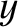plane.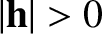Next: Plane polar coordinates Up: Keplerian orbits Previous: Kepler's laws
Richard Fitzpatrick 2016-03-31